# FTCE Properties of Equality & Operations Flashcards

FTCE Properties of Equality & Operations Flashcards
1/44 (missed) 0 0
Create Your Account To Continue Studying

As a member, you'll also get unlimited access to over 79,000 lessons in math, English, science, history, and more. Plus, get practice tests, quizzes, and personalized coaching to help you succeed.

Try it risk-free for 30 days. Cancel anytime
Identify the property represented by: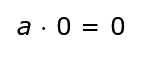Multiplication Property of Zero
Got it
Identify the formula for the reflexive property of equality.
The formula is: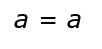Got it
Identify the property of the commutative property of multiplication.
The formula is: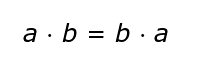Got it
Identify the formula for the multiplication property of equality.
The formula is: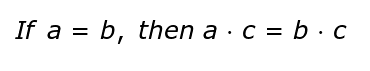Got it
Identify the formula for the subtraction property of equality.
The formula is: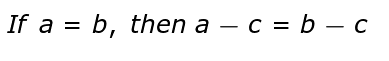Got it
Identify the formula for the symmetric property.
The formula is: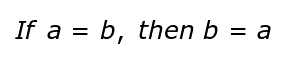Got it
Identify the property represented by: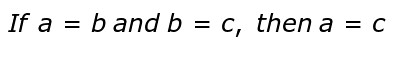Transitive Property of Equality
Got it
Identify the property represented by: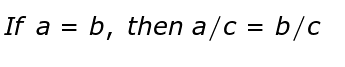Division Property of Equality
Got it
Identify the property represented by: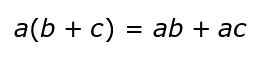Distributive Property
Got it
Identify the property represented by: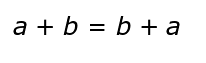Got it
Identify the property represented by: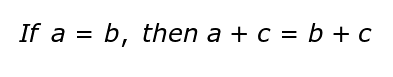Got it
Division Property of Equality
If you divide one side of the equation by something, then you must divide the other side by the same thing to keep the equation the same.
Got it
Multiplication Property of Equality
If you multiply one side of the equation by something, then you must multiply the other side by the same thing to keep the equation the same.
Got it
Subtraction Property of Equality
If you subtract something from one side of the equation, then you must subtract the same thing from the other side to keep the equation the same.
Got it
If you add something to one side of the equation, then you must add the same thing to the other side to keep the equation the same.
Got it
Reflexive Property of Equality
Any term is equal to itself.
Got it
Distributive Property
Multiply the number on the outside of the parentheses with each term on the inside of the parentheses.
Got it
Multiplication Property of Zero
The product of a term and zero is zero.
Got it
Commutative Property of Multiplication
In a multiplication sentence, you can multiply the numbers in any order and still get the same answer.
Got it
In an addition sentence, you can add the numbers in any order and still get the same answer.
Got it

or choose a specific lesson: See all lessons in this chapter
44 cards in set

## Flashcard Content Overview

There is typically more than one way to write the same equation. These are called equivalent equations and they allow students to approach a problem in way that may help them solve it more easily. But what makes one equation equivalent and not another? The properties of equality and operations set the rules for creating equivalent equations. Most young students learn how to apply the properties far before they actually learn their names and definitions, but as a teacher, you need to understand the building blocks so you can teach the students appropriately. This flashcard set is a great overview of eleven of the properties of equality and operations. You will find a card that tests your knowledge of the definition and the formula for each property. There are also two application based cards for each that cover property identification, property within an equation or expression and using a given property to solve for an unknown. If you find that you struggle with any of the individual properties, be sure to review those lessons accordingly.

Front
Back
In an addition sentence, you can add the numbers in any order and still get the same answer.
Commutative Property of Multiplication
In a multiplication sentence, you can multiply the numbers in any order and still get the same answer.
Multiplication Property of Zero
The product of a term and zero is zero.
Distributive Property
Multiply the number on the outside of the parentheses with each term on the inside of the parentheses.
Reflexive Property of Equality
Any term is equal to itself.
If you add something to one side of the equation, then you must add the same thing to the other side to keep the equation the same.
Subtraction Property of Equality
If you subtract something from one side of the equation, then you must subtract the same thing from the other side to keep the equation the same.
Multiplication Property of Equality
If you multiply one side of the equation by something, then you must multiply the other side by the same thing to keep the equation the same.
Division Property of Equality
If you divide one side of the equation by something, then you must divide the other side by the same thing to keep the equation the same.
Identify the property represented by:Identify the property represented by:Identify the property represented by:Distributive Property
Identify the property represented by:Division Property of Equality
Identify the property represented by:Transitive Property of Equality
Identify the formula for the symmetric property.
The formula is:Identify the formula for the subtraction property of equality.
The formula is:Identify the formula for the multiplication property of equality.
The formula is:Identify the property of the commutative property of multiplication.
The formula is:Identify the formula for the reflexive property of equality.
The formula is:Identify the property represented by:Multiplication Property of Zero
Transitive Property of Equality
If two terms are equal to one another and the second term is also equal to a third term, then the first term is equal to the third term as well.
Symmetric Property
This property allows you to exchange the left side and the right side of an equation and have it remain equal.
Identify which property is represented by the following: 8 + 10 = 10 + 8
Identify which property is represented by the following: -2(x + 3) = -2x - 6
Distributive Property
Identify which property is represented by the following: -9 = -9
Reflexive Property

Identify the property that is represented by the following:

x - 8 = 10

x -8 + 8 = 10 + 8

x = 18

Identify the property that is represented by the following: 4 x 5 x 2 = 5 x 2 x 4
Commutative Property of Multiplication
Identify the property that is represented by the following: 18 x 0 = 0
Multiplication Property of Zero

Identify the property that is represented by the following:

9 + x = 15

9 + x - 9 = 15 - 9

x = 6

Subtraction Property of Equality

Identify the property that is represented by the following:

a/5 = 7

a/5 x 5 = 7 x 5

a = 35

Multiplication Property of Equality

Identify the property that is represented by the following:

9x = -72

9x/9 = -72/9

x = -8

Division Property of Equality
Identify the property that is represented by the following: If x = y and y = 10, then x = 10
Transitive Property of Equality
Identify the property that is represented by the following: b = -10 then -10 = b
Symmetric Property of Equality
Use the distributive property to simplify this equation: 4(c - 5) =
4c - 20
Use the commutative property of addition to write an equivalent form of this expression: 2 + 11
11 + 2
Use the reflexive property of equality to find what x equals in the following: x = 13 + 15
28
Use the addition property of equality to find that k equals: k - 12 = 22
k = 34
Use the subtraction property of equality to find what m equals: 10 + m = 44
m = 34
Use the multiplication property of equality to find what x equals: x/-3 = -8
x = 24
Use the division property of equality to find what y equals: 4y = -36
y = -9
Use the commutative property of multiplication to write an equivalent form of the following expression: 2 x 13
13 x 2
Use the multiplication property of zero to find the solution to the following equation: 1,456 x 0 =
0
Use the transitive property of equality to fill in the blank with the appropriate number: If x = y and y = -14, then x =___
-14
Write an equivalent form of the equation using the symmetric property: 4 = 16 - 12
16 - 12 = 4

To unlock this flashcard set you must be a Study.com Member.